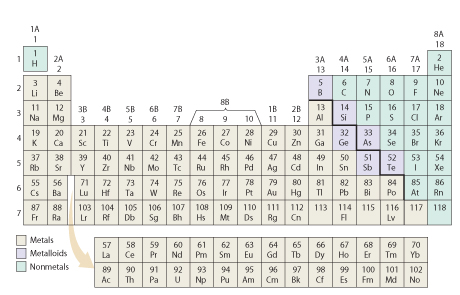# Problem: While it is helpful to know that many ions have the same number of electrons as a noble gas, many elements, especially among the metals, form ions that do not have the same number of electrons as a noble gas.Use the periodic table shown below, to determine which of the following ions have the same number of electrons as a noble gas.Check all that apply.a) Zn 2+ b) Te2−c) Ti4+d) Mn2+ e) Pb2+

###### FREE Expert Solution

It can be deduced that some atoms tend to form ions to acquire the number of electrons of the noble gases because the electron arrangements with these numbers of electrons are very stable.

• Ti → atomic # = 22
89% (57 ratings)###### Problem Details

While it is helpful to know that many ions have the same number of electrons as a noble gas, many elements, especially among the metals, form ions that do not have the same number of electrons as a noble gas.

Use the periodic table shown below, to determine which of the following ions have the same number of electrons as a noble gas.Check all that apply.

a) Zn 2+

b) Te2−

c) Ti4+

d) Mn2+

e) Pb2+

Frequently Asked Questions

What scientific concept do you need to know in order to solve this problem?

Our tutors have indicated that to solve this problem you will need to apply the Ions concept. You can view video lessons to learn Ions. Or if you need more Ions practice, you can also practice Ions practice problems.

What professor is this problem relevant for?

Based on our data, we think this problem is relevant for Professor Morgan's class at ASU.